﻿ Flow around a rotating disk - XSim

# Flow around a rotating disk

Update: June 1, 2017
OpenFOAM 4.x

## Case directory

\$FOAM_TUTORIALS/incompressible/simpleFoam/rotorDisk

## Summary

We calculate the flow velocity and pressure around a rotating disk placed in the flow.

Fluid flows in from the region "inlet" (red part) at (0, 1, 0) m/s, flows out from the region "outlet" (blue part), and the region "rotatingZone" (green part) rotates at 1000 rpm with the Y-axis as the axis of rotation. The wall of the cylinder is set to the slip wall condition.Model geometry

The rotation axis, rotation speed, etc. are set in the file system/fvOptions for the cell zone "rotatingZone". The k-ω SST model is used as the turbulence model.

The meshes are as follows, and the number of mesh is 145265.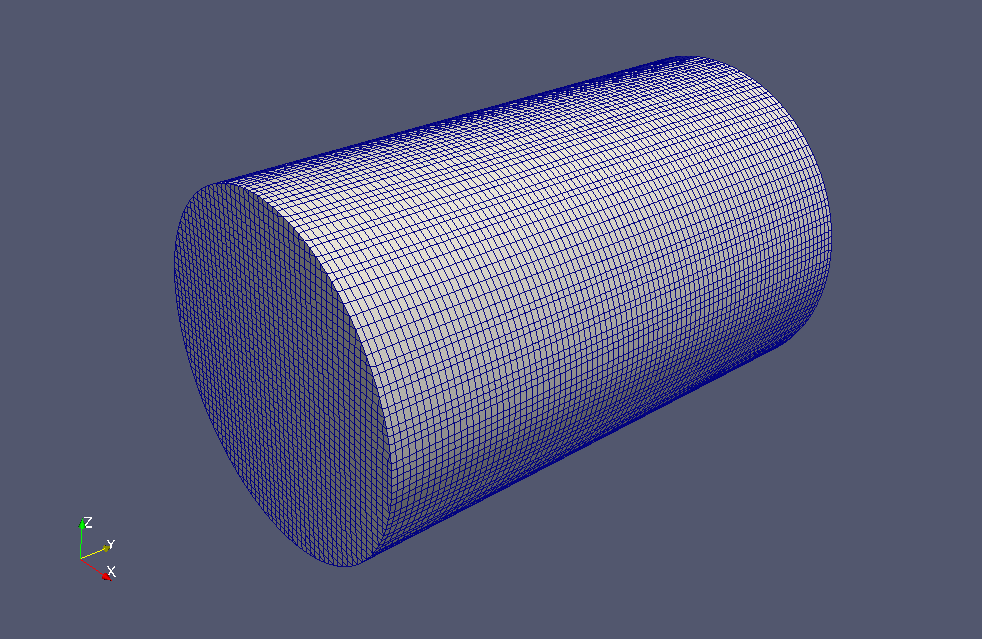Mesh (entire)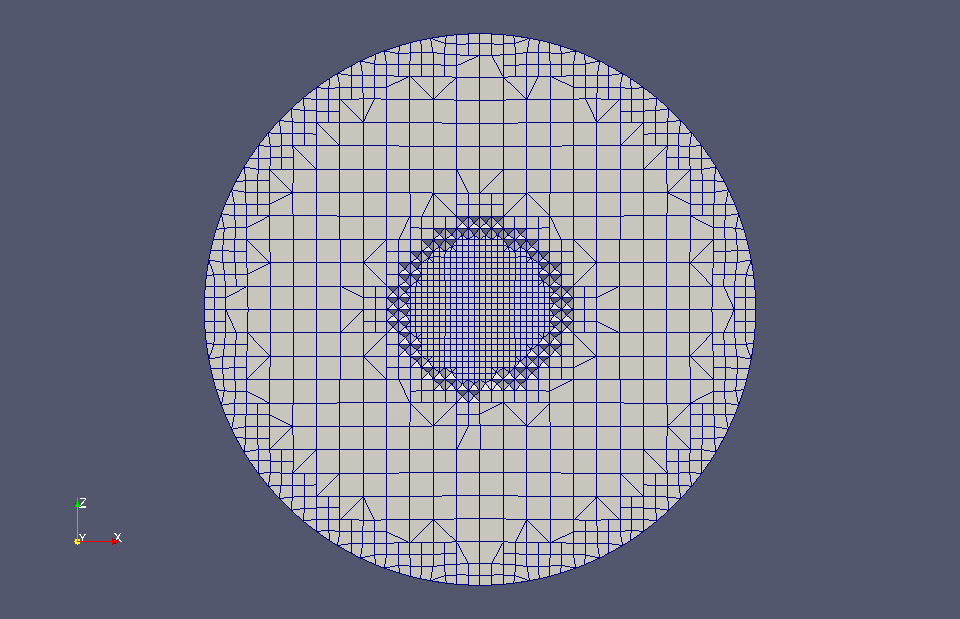Mesh (at XZ-plane)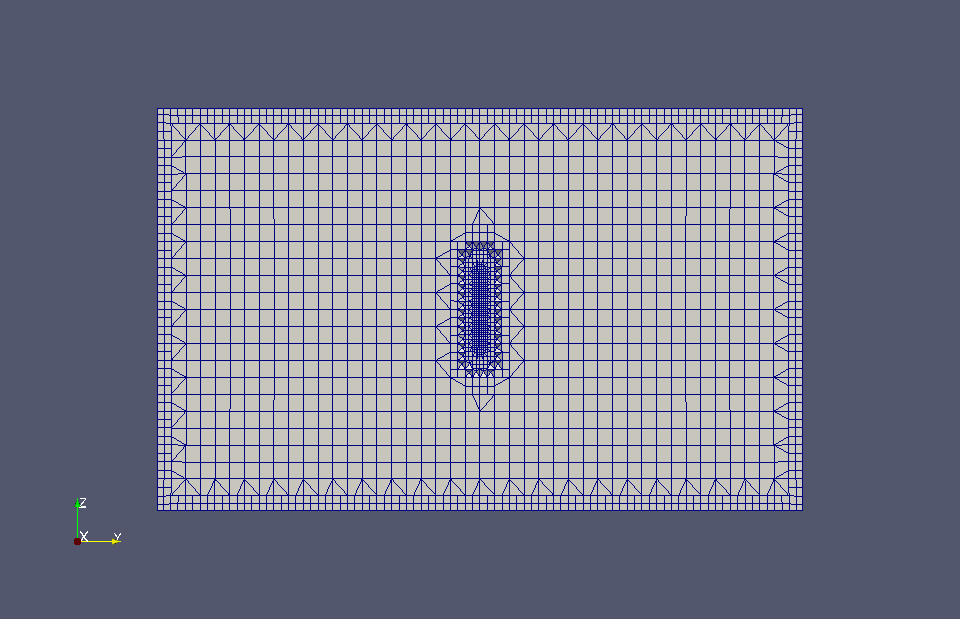Mesh (at YZ-plane)

To visualize the calculated turbulence energy and specific turbulence dissipation rate, check "k" and "omega" in the "Properties" tab in ParaView.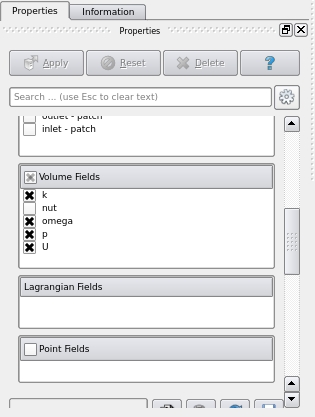Check "k" and "omega"

The calculation results in the main flow direction are as follows.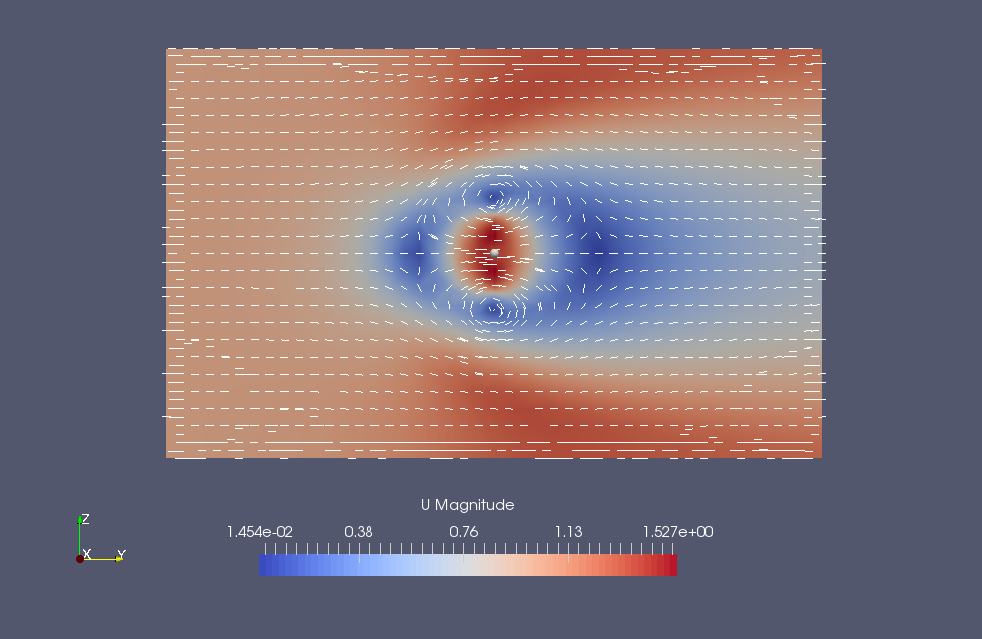Flow velocity at YZ-plane (U)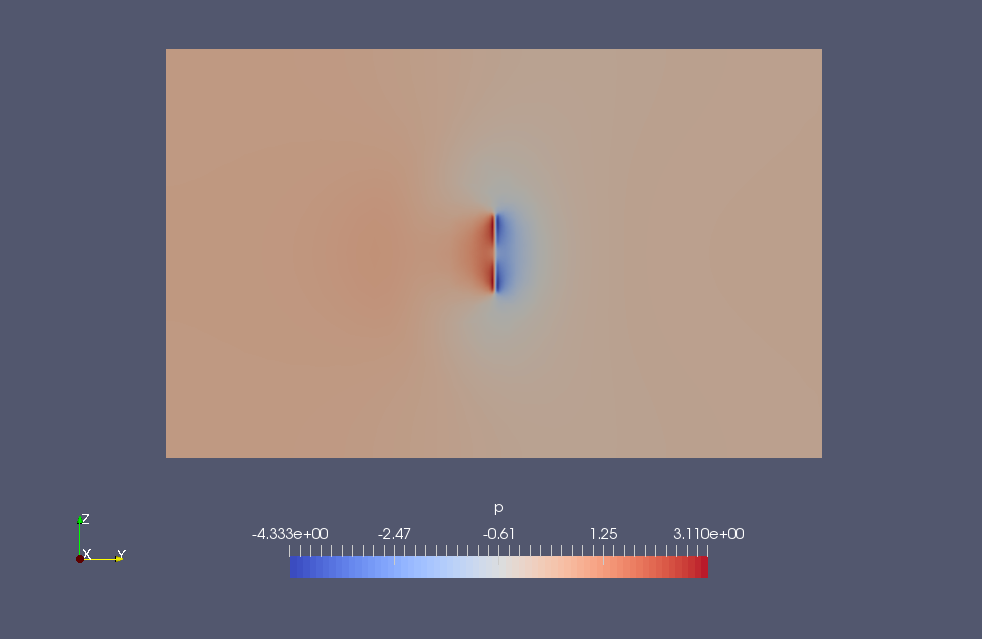Pressure at YZ-plane (p)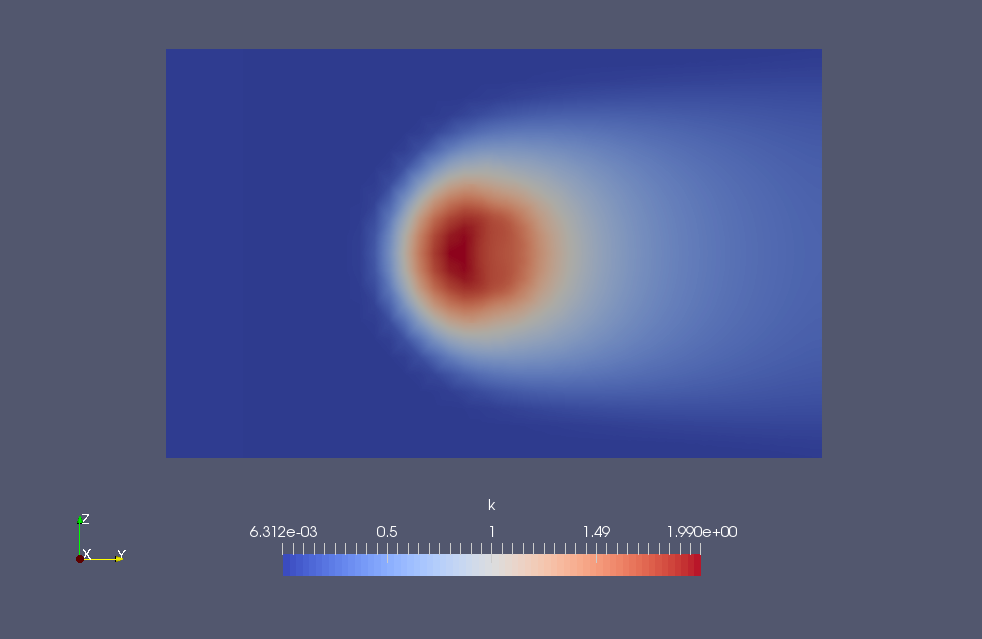Turbulence energy at YZ-plane (k)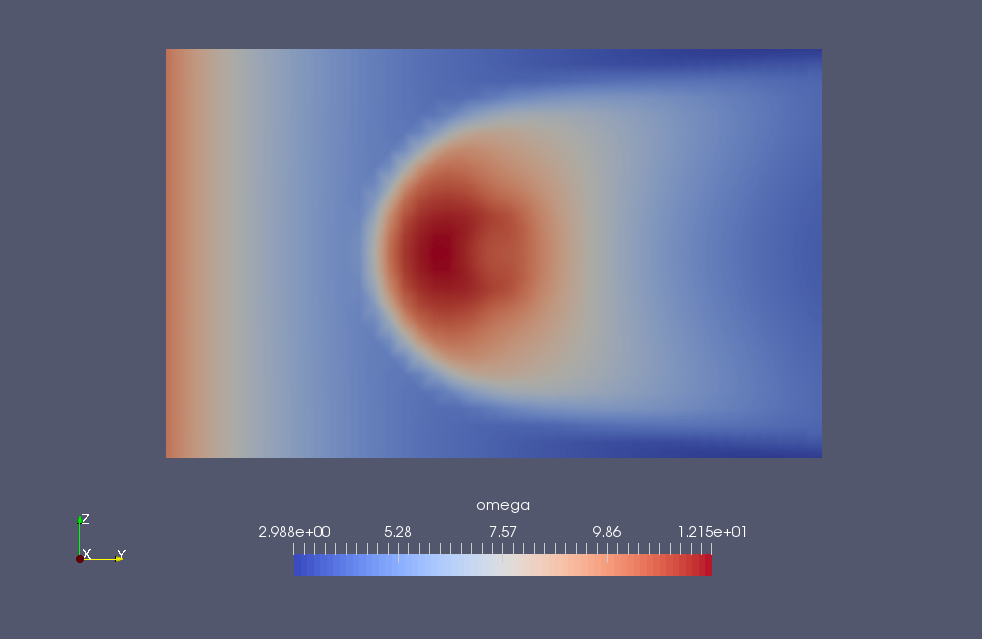Specific turbulence dissipation rate at YZ-plane (omega)

The calculation results in the direction parallel to the rotating disk are as follows.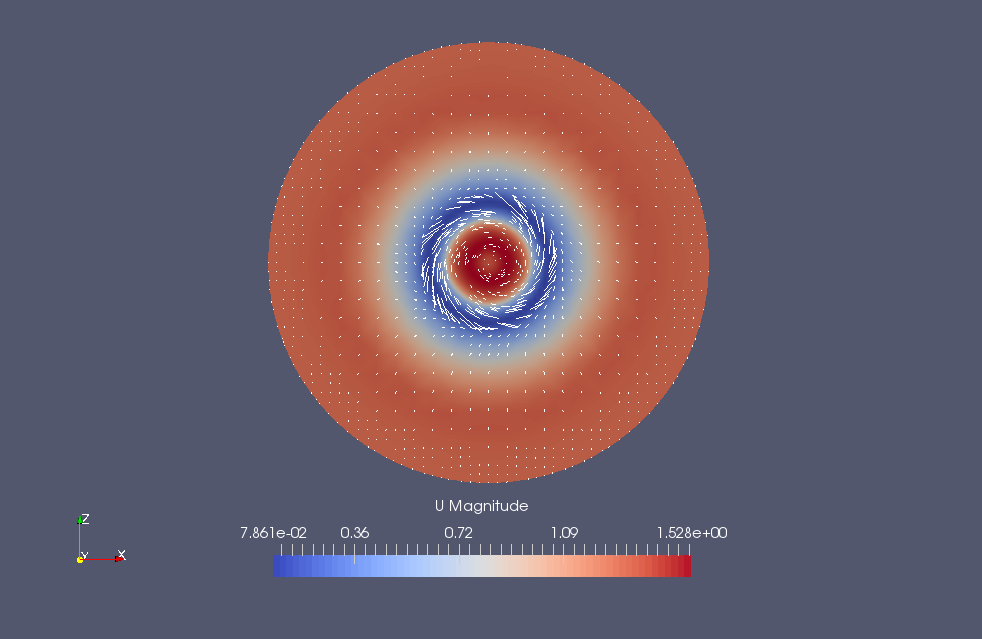Flow velocity at XZ-plane (U)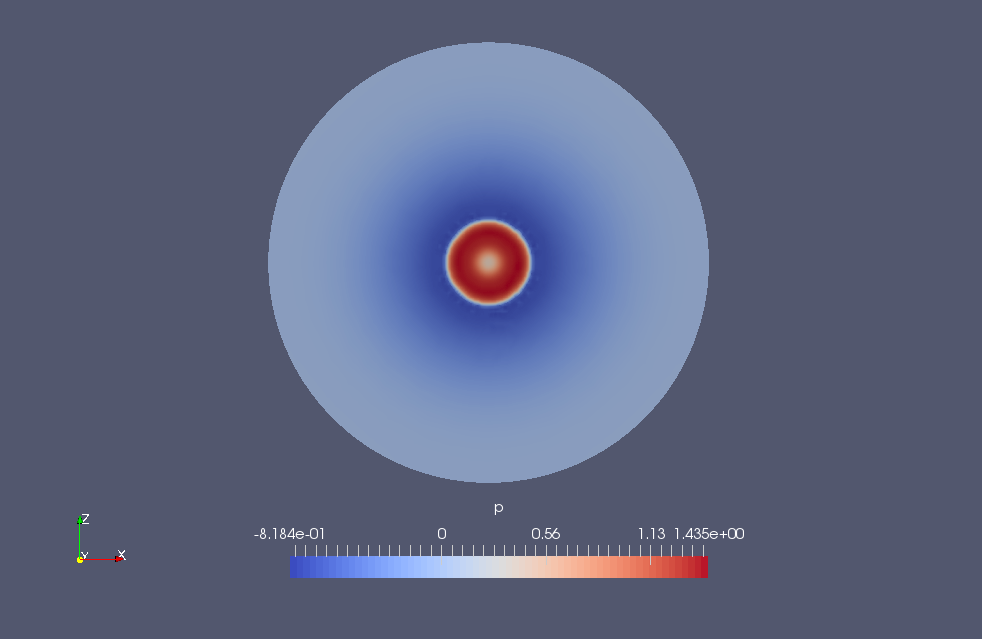Pressure at XZ-plane (p)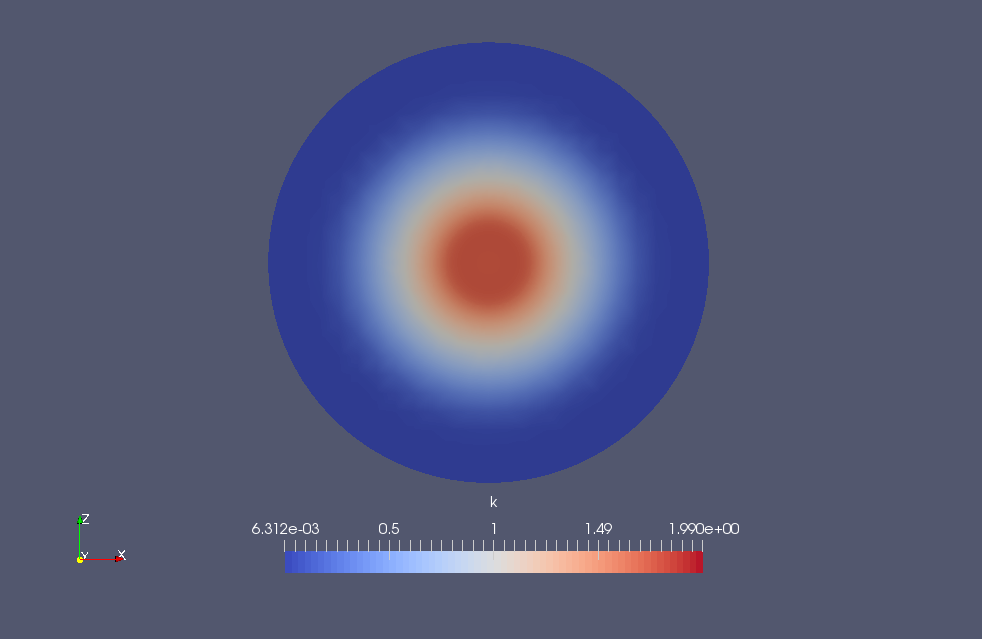Turbulence energy at XZ-plane (k)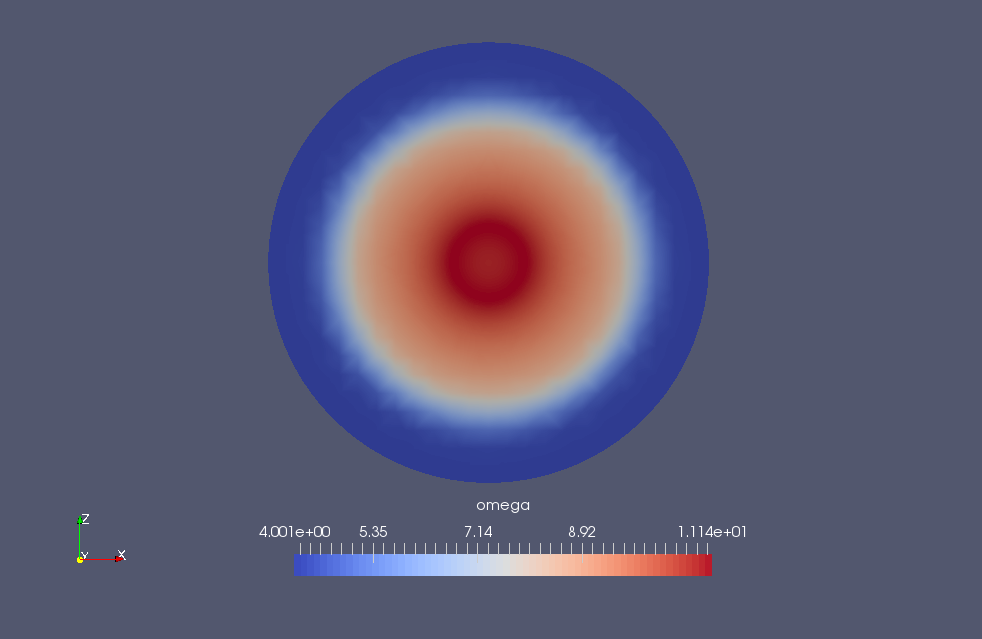Specific turbulence dissipation rate at XZ-plane (omega)

## Commands

cp -r \$FOAM_TUTORIALS/incompressible/simpleFoam/rotorDisk rotorDisk
cd rotorDisk

blockMesh
surfaceFeatureExtract
snappyHexMesh -overwrite

simpleFoam

paraFoam

Based on the shape files (fixed.obj and rotatingZone.obj) in the directory constant/triSurface, the meshes are created with the blockMesh, surfaceFeatureExtract, and snappyHexMesh utilities.

## Calculation time

106.27 seconds *Single, Inter(R) Core(TM) i7-2600 CPU @ 3.40GHz 3.40GHz